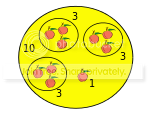0

## The Great Divide II

Published on Sunday, May 04, 2014 in , , ,My previous post, The Great Divide, focused on divisibility testing for numbers ending in 9, such as 19, or for numbers which could be scaled up to a number ending in 9, such as 13, which can be scaled up to 39.

The tests took advantage of the fact that they have neighboring numbers ending in 0, which are easy to divide by, and can easily generate a quotient and remainder.

Assuming you're familiar and comfortable with the previous approach, today's post will focus on divisibility testing for numbers ending in 1.

DIVISIBILITY BY 11: In the previous post, we saw that we could test for divisibility by 11 through scaling it up to 99, and taking advantage of the ease of dividing by 100.

For example, to check whether 1,353 is divisible by 11, we'd divide 1,353 by 100, giving us a quotient of 13 and a remainder of 53. Since 13 + 53 = 66, and 66 is obviously a multiple of 11, we can see that 1,353 is evenly divisible by 11.

However, since 11 is right next to 10, isn't there a way to take advantage of that relationship without scaling 11? Yes, there is!

Just as before, we divide by the neighbor to get a remainder and a quotient, but this time we subtract (instead of adding) the smaller of the two numbers from the larger of the two numbers.

Let's try this new version of the test on 1,353 again. Dividing 1,353 by 10, we get 135 remainder 3. This time, we need to subtract the smaller number from the larger, so we work out 135 - 3 = 132.

If you know enough multiples of 11, you should recognize 132 as a multiple of 11. If not, just as with the tests for numbers ending in 9, you can repeat the test on the new number. 132 ÷ 10 = 13 remainder 2, and 13 - 2 = 11, so 1,353 (and 132, for that matter) is confirmed as a multiple of 11.

HOW CLOSE ARE WE TO A MULTIPLE?: Also, just as before, numbers that don't pass these tests for divisibility can still show how close they are to a multiple, even through repeated testing.

As an example, let's test 4,085 for divisibility by 11. 4,085 ÷ 10 = 408, remainder 5, and 408 - 5 = 403. Testing 403 for divisibility by 11, we work out 403 ÷ 10 = 40, remainder 3, and 40 - 3 = 37. We know 37 isn't evenly divisible by 11, so 4,085 isn't evenly divisible by 11.

Since 37 is only 4 more than 33, which is a multiple of 11, we can also say that 4,085 must be 4 more than a multiple of 11. 4,085 - 4 = 4,081, and we can verify that 4,081 is a multiple of 11 by our own testing, or with the help of Wolfram|Alpha.

WHY DOES THIS WORK?: This works for similar reasons as the previous method works. In this case, we're testing a number x for divisibility by n by using n - 1 to get a quotient, q, and remainder, r, so this version looks like this:

x(n - 1) = q - r

Solving this equation for x, we get:

x = (n - 1)(q - r)

Expanding this works out to:

x = nq - nr - q + r = nq - nr - (q - r)

From here, the logic is basically the same as in my previous post. Since each of the variables are defined as integers, nq - nr must be evenly divisible by n. Therefore, as long as subtracting q - r is evenly divisible by n, then x must be evenly divisible by n.

TESTING OTHER NUMBERS BY SCALING: Just as with the approach in my previous post, numbers can be scaled to work with this approach. For example, in the previous post we scaled 7 up to 49, so we could test for divisibility using 50.

Thanks to the new version of the test for numbers ending in a 1, we can scale 7 up to 21, and use 20 for testing instead.

Is 2,667 evenly divisible by 7? Let's use the scaling up to 21, so we can divide by 20 in our tests. 2,667 ÷ 20 = 133, remainder 7, and 133 - 7 = 126. Personally, I know enough multiples of 126 to recognize that it's a multiple of 7. What if I didn't?

I'd just test 126 again. 126 ÷ 20 = 6, remainder 6, and 6 - 6 = 0. Yes, 0 counts as a multiple of 7, so 2,667 is evenly divisible by 7.

WIDE VARIETY OF OPTIONS: With this new version of divisibility testing, you can now test for divisibility by any number ending in 9 or 1. Further, you can multiply any number ending in 3 by 3 to get a number ending in 9, or any number ending in 7 by 3 to get a number ending in 1. So, you've got a wide variety of tests for any number ending in 1, 3, 7, or 9 that you can recall how to do with a little practice and effort!

When testing for primes, this gives you an arsenal of tests that you can keep straight and use quickly and easily. Testing for divisibility by 11, 19, 29, and so on? No problem, as they're ready made for the test! Need to test for divisibility by 23? Scale it up to 69, and use 70 in your tests! Divisibility by 17? Scale it up to 51, and use 50 in your tests!

This is a little unusual when it's a new concept, but continued practice will give you a tool well worth having in your mathematical arsenal.